##### Electronics For DummiesYou can combine capacitors in series or parallel networks to create any capacitance value you need in an electronic circuit. For instance, if you combine three 100 μF capacitors in parallel, the total capacitance of the circuit is 300 μF.

## Combine capacitors in parallel

Calculating the total capacitance of two or more capacitors in parallel is simple: Just add up the individual capacitor values to get the total capacitance.

This rule makes sense if you think about it for a moment. When you connect capacitors in parallel, you're essentially connecting the plates of the individual capacitors. So connecting two identical capacitors in parallel essentially doubles the size of the plates, which effectively doubles the capacitance.

Here, the two circuits have identical capacitances. The first circuit accomplishes the job with one capacitors, the second does it with three. Thus, the circuits are equivalent.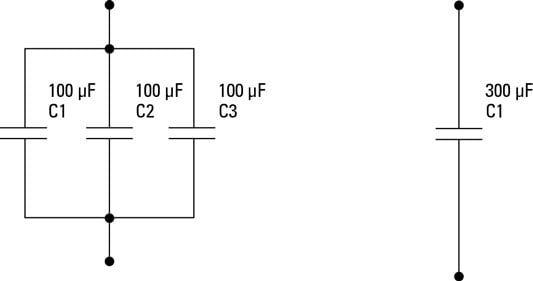Whenever you see two or more capacitors in parallel in a circuit, you can substitute a single capacitor whose value is the sum of the individual capacitors. Similarly, any time you see a single capacitor in a circuit, you can substitute two or more capacitors in parallel as long as their values add up to the original value.

The total capacitance of capacitors in parallel is always greater than the capacitance of any of the individual capacitors. That's because each capacitor adds its own capacitance to the total.

## Connect capacitors in series

You can also combine capacitors in series to create equivalent capacitances. When you do, however, the math is a little more complicated. It turns out that the calculations required for capacitors in series are the same as calculating resistors in parallel.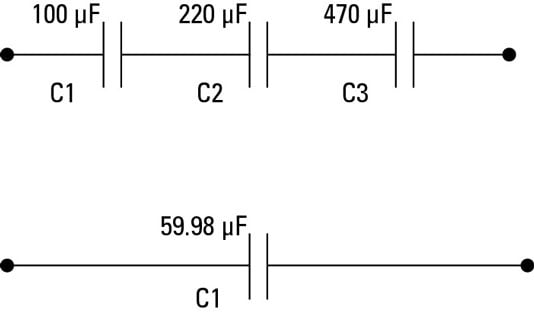Here are the rules for calculating capacitances in series:

• If the capacitors are of equal value, you're in luck. All you must do is divide the value of one of the individual capacitors by the number of capacitors. For example, the total capacitance of two, 100 μF capacitors is 50 μF.

• If only two capacitors are involved, use this calculation: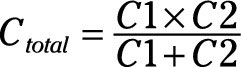In this formula, C1 and C2 are the values of the two capacitors.

Here's an example, based on a 220 μF and 470 μF capacitor in series: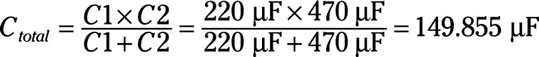• For three or more capacitors in series, the formula is this: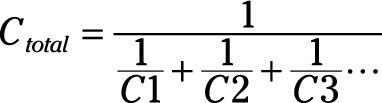Note that the ellipsis at the end of the expression indicates that you keep adding up the reciprocals of the capacitances for as many capacitors as you have.

Here is an example for three capacitors whose values are 100 μF, 220 μF, and 470 μF: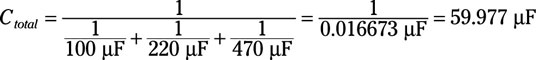As you can see, the final result is 59.9768 μF. Unless your name happens to be Spock, you probably don't care about the answer being so precise, so you can safely round it to an even 60 μF.

The formulas for calculating the total capacitance of a capacitor network are the reverse of the rules you follow to calculate resistor networks. In other words, the formula you use for resistors in series applies to capacitors in parallel, and the formula you use for resistors in parallel applies to capacitors in series. Isn't it funny how science sometimes likes to mess with your mind?